# ROUNDDOWN Excel FunctionArticle byExcelMojo Team## What Is ROUNDDOWN Function In Excel?

The ROUNDDOWN function in Excel rounds down the numbers in a dataset to the nearest hundreds place, tens place, to the left of the decimal point, or to the required number of decimal places, to the right of the decimal point. The ROUNDDOWN Excel function is an inbuilt function, so we can enter the formula directly in the worksheet or insert it from the “Function Library”.

For example, the table below contains numbers in column A. We will apply the ROUNDDOWN Excel function as per the requirements in column B. Accordingly, column C provides the number of digits the given values should get rounded down to for achieving the required data in column D.

Select cell D2, enter the formula =ROUNDDOWN(A2,C2), and press the “Enter” key. Then, drag the formula from cell D2 to D8 using the fill handle.

We get the output as shown above, in column D. Column E is for our reference.

[Output Observation: Let us consider the target cell D4 formula. The ROUNDDOWN Excel function is 3.141592, and returns the rounded-down number as 3.1415. The reason is that the requirement is to round down the value, 3.141592, to four decimal places.]

###### Key Takeaways
• The ROUNDDOWN Excel function rounds down the given real number to the specified number of digits, towards zero, which improves the data accuracy of their datasets.
• While each argument value can be a single number or an expression returning a number, we can supply the arguments to the function as array ranges. However, in such a case, we will execute the formula using the shortcut keys Ctrl + Shift + Enter.
• We can use the ROUNDDOWN() with other Excel functions such as IF and VLOOKUP.

### ROUNDDOWN() Excel Formula

The ROUNDDOWN Excel formula syntax is:

The ROUNDDOWN Excel formula arguments are:

• number: The cell or cell range containing the numeric values to be rounded. It is a mandatory argument.
• num_digits: The number of digits to round down the given number to. It is a mandatory argument.

### How To Use ROUNDDOWN Excel Function?

We can use the ROUNDDOWN Excel Function in 2 methods, namely,

1. Access from the Excel ribbon.
2. Enter in the worksheet manually.

#### Method #1 – Access from the Excel ribbon

First, choose an empty cell for the output → select the “Formulas” tab → go to the “Function Library” group → click the “Math & Trig” option drop-down → select the “ROUNDDOWN” function, as shown below.

The “Function Arguments” window pops up. Enter the arguments in the “Number” and “Num_digits” fields → click “OK”, as shown below.

#### Method #2 – Enter in the worksheet manually

The steps to apply the ROUNDDOWN Excel function are:

1. Select an empty cell for the output.
2. Type = ROUNDDOWN( in the cell. [Alternatively, type =R and double-click the ROUNDDOWN function from the Excel suggestions.]
3. Enter the argument values and close the brackets.
4. Finally, press the “Enter” key.

Let us an example to round down the given price values to two decimal places using ROUNDDOWN Excel function.

The following table contains a grocery list and the price details in columns A and B.

The steps to round a number using ROUNDDOWN Excel function are:

1. Select cell C2, enter the formula =ROUNDDOWN(B2,2), and press the “Enter” key.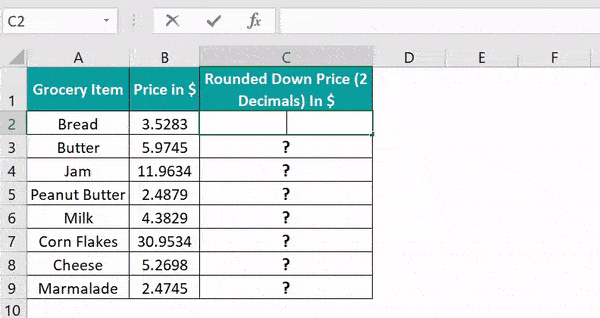[Alternatively, select cell C2 and apply the ROUNDDOWN() from the FormulasMath & TrigROUNDDOWN. It will open the Function Arguments window.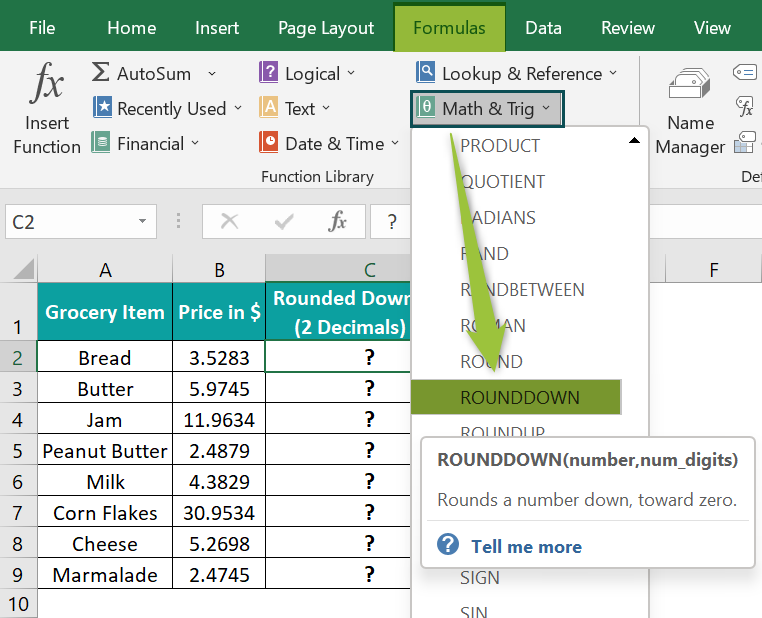Enter the two argument values in the Function Arguments window, as shown below.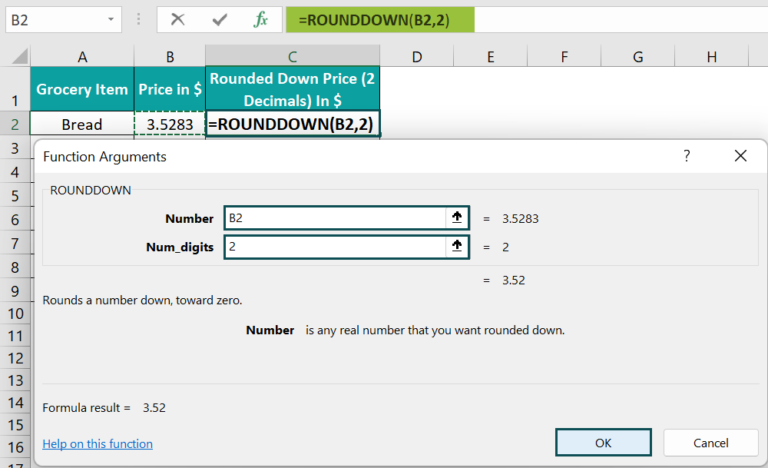Finally, when we click OK, the formula gets executed for 2 decimal places in the target cell.]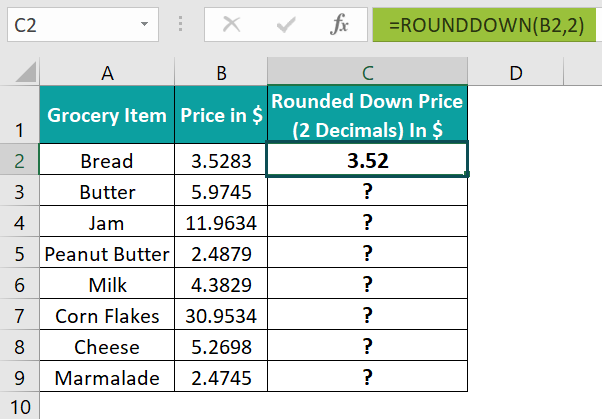2. Drag the formula from cell C2 to C9 using the fill handle.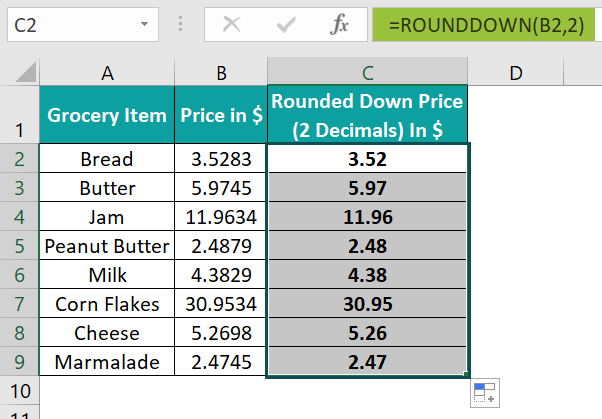The output is shown above.

[Output Observation: The formula in cell C9 works as follows, num_digits argument is 2, a value greater than 0, the given number 2.4745 gets rounded down to two decimal places, 2.47.]

### Examples

We can understand some advanced scenarios using the ROUNDDOWN Excel function examples.

#### Example #1

We will use the ROUNDDOWN Excel function when the number we require to round down is a negative value.

The below table contains the details of companies with negative one-day changes in share values and the “num_digits”.

The steps to use the ROUNDDOWN Excel function are as follows:

• Step 1: Select cell D2, enter the formula =ROUNDDOWN(B2,C2), and press the “Enter” key.
• Step 2: Drag the formula from cell D2 to D6 using the fill handle.

We get the output as shown above.

[Output Observation: In row 1, the given value, -0.85, gets rounded down to 1 decimal place and becomes -0.8. In row 2, the value, -11.26, gets rounded down to the nearest 10th place, and the result we see is -10. And in row 3, the number -2.54 gets rounded down to two decimal places. But, as the number is already a value with two decimal places after the decimal point, it remains unchanged even after applying the formula.

On the other hand, looking at the result in row 5, it might appear that the ROUNDDOWN Excel function does not work for a negative integer and 0 num_digits. However, in this example, the second argument is 0, which rounds down the given number to the nearest integer. And as the specified number is already an integer, -2, it remains the same even after rounding down.

Finally, in row 6, the given value, -3.73, needs to get rounded down to the nearest 100th place. However, the value has only one digit before the decimal point. Hence, the number gets rounded down to 0. Had the number been, for instance, -350.73, it would get rounded down to -300.]

#### Example #2

We will use the ROUNDDOWN Excel function to round down the result of an expression, evaluating to a number.

The following table contains two sets of values. And column D shows the results of the mathematical operations without rounding down.

The steps to use the ROUNDDOWN Excel function are as follows:

• Step 1: First, select cell E2, enter the formula =ROUNDDOWN((A2+B2),0), and press the “Enter” key.
• Step 2: Next, select cell E3, enter the formula =ROUNDDOWN((A3-B3),0), and press the “Enter” key.
• Step 3: Then, select cell E4, enter the formula =ROUNDDOWN((A4*B4),0), and press the “Enter” key.
• Step 4: Finally, select cell E5, enter the formula =ROUNDDOWN((A5/B5),0), and press the “Enter” key.

We get the output for the specified rounding as shown above.

#### Example #3

We will use the ROUNDDOWN Excel function with other functions such as IF and VLOOKUP.

The below table shows a list of fruits and their quantity details, and we want to round down the quantity value of a given fruit in cell E2 to the nearest 10th place.

The procedure to use the ROUNDDOWN with VLOOKUP and IF Excel Function is:

• Step 1: Select cell F2, enter the formula =IF(VLOOKUP(E2,A:B,2,0),ROUNDDOWN(VLOOKUP(E2,A:B,2,0),-1),”No Data”), and press the “Enter” key.

We get the output as shown above.

[Output Observation: Here, the VLOOKUP() looks up the quantity value for the specified fruit, watermelon, and returns the value 195.4201. And as the IF condition is a value, translating as TRUE, the ROUNDDOWN() executes. It rounds down the quantity of watermelon (looked up from the first table) to the nearest 10th place to return the value, 190.]

### Important Things to Note

• If the num_digits value is less than zero, the ROUNDDOWN Excel function rounds down the given value to the left of the decimal point.
• When the num_digits value is equal to zero, the given number gets rounded down to the nearest integer.
• For a num_digits value greater than zero, the ROUNDDOWN() rounds down the given number to a specific number of decimal places.

1. Where is the ROUNDDOWN function in Excel?

The ROUNDDOWN function in Excel is in the FormulasMath & TrigROUNDDOWN.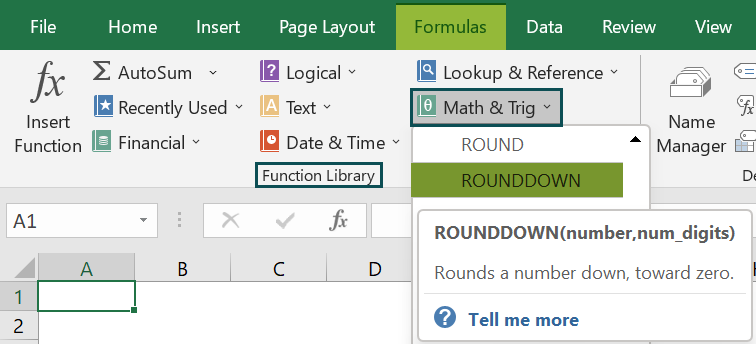2. How to use the ROUNDDOWN function in Excel VBA?

We can use the ROUNDDOWN() in VBA using the WorksheetFunction.RoundDown() method.

For example, the following table contains a list of numbers and the corresponding number of places to which we require to round them down.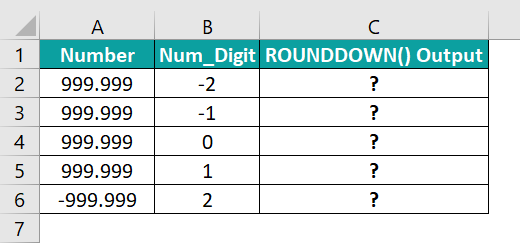The steps to use ROUNDDOWN() in VBA are:

• 1: In the worksheet containing the table, press the keys Alt + F11 to open the VBA Editor.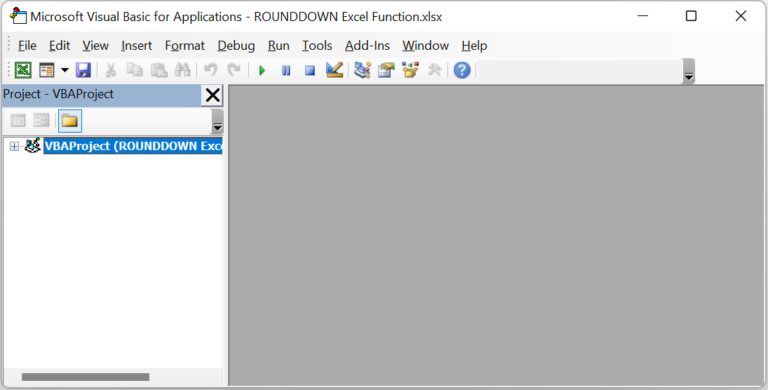• 2: Choose the required VBAProject in the VBA Editor window and select InsertModule to open a new module window, Module1.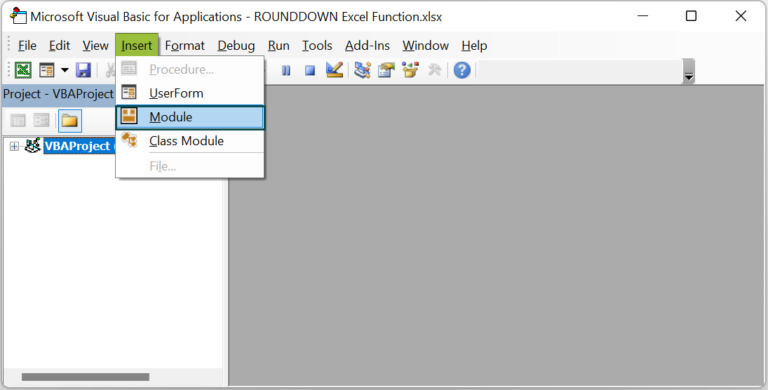• 3: Enter the VBA code, as shown in the image below, in the Module1 window.

Sub ROUNDDOWN_fn()
Dim rd As Worksheet
Set rd = Worksheets(“FAQ 2”)
rd.Range(“C2”) = Application.WorksheetFunction.Rounddown(rd.Range(“A2”), rd.Range(“B2”))
rd.Range(“C3”) = Application.WorksheetFunction.Rounddown(rd.Range(“A3”), rd.Range(“B3”))
rd.Range(“C4”) = Application.WorksheetFunction.Rounddown(rd.Range(“A4”), rd.Range(“B4”))
rd.Range(“C5”) = Application.WorksheetFunction.Rounddown(rd.Range(“A5”), rd.Range(“B5”))
rd.Range(“C6”) = Application.WorksheetFunction.Rounddown(rd.Range(“A6”), rd.Range(“B6”))
End Sub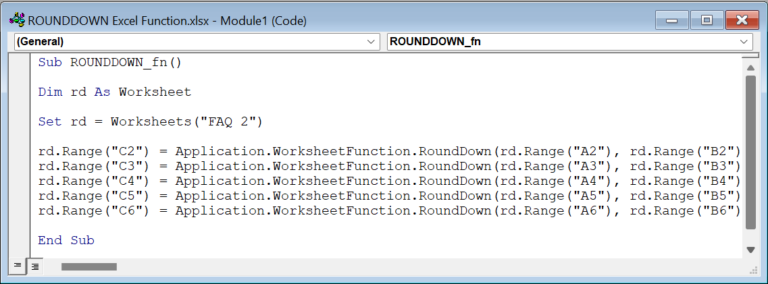• 4: Click the Run Sub/UserForm icon to execute the Module1 code.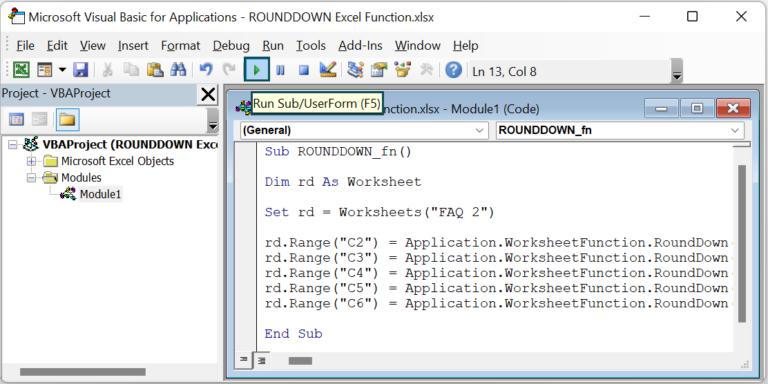Now open the active worksheet to view the required rounded-down numbers in the specified target cells. The output is shown below.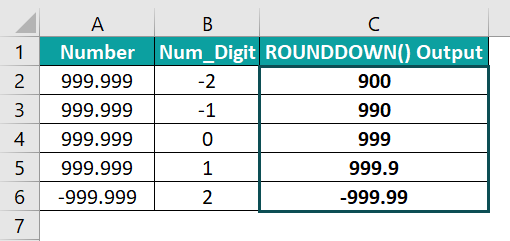3. Why is the Excel ROUNDDOWN function not working?

The ROUNDDOWN Excel function may not work for the following reasons:
If the supplied arguments are not numbers or expressions evaluating to numbers.
The column width is insufficient to show the complete rounded-down number.

4. What is the difference between the ROUNDDOWN and FLOOR Excel functions?

The difference between the ROUNDDOWN and FLOOR Excel functions is that the ROUNDDOWN() rounds the given number down to the specified number of places. On the other hand, the FLOOR() also rounds down the given number but to the closest multiple of the specified significance.